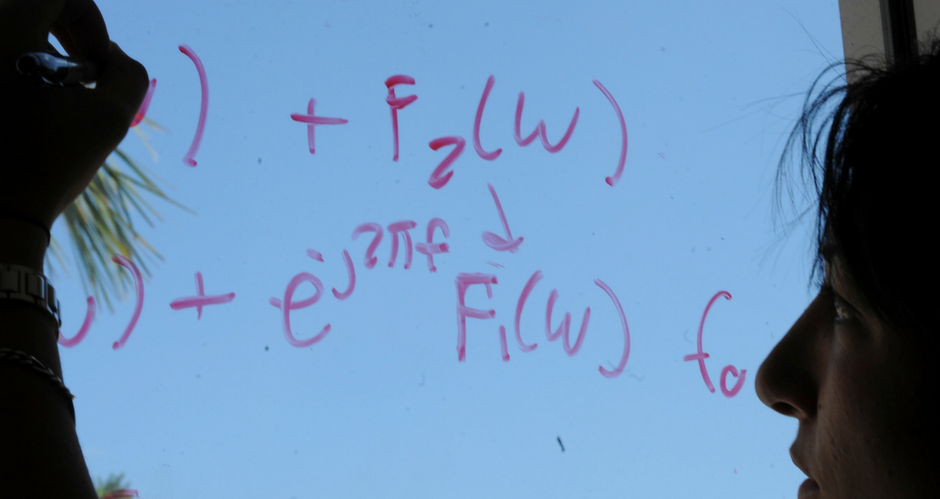## Mathematics and System Engineering Student Publications

### Applications of Random Matrices to Image Processing for Image Denoising

Poster

#### Abstract

Random matrices were first introduced in studies related to mathematical statistics by Wishart, Hsu. Later, Dyson recognized that would be required a new type of statistical mechanics to describe the nuclear energy levels and Wigner in his seminal work modelling the spectra of heavy atoms found that random matrices were the answer to this problem. Moreover, Wigner stated his famous semicircle law, which describes the eigenvalue density for a gaussian ensemble of random matrices as depicted in Fig. 1. The goal here is to use random matrix theory for applications to image processing.

2015